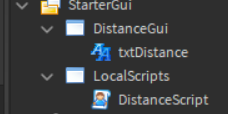# Help finding distance from HumanoidRootPart to a part and printing on TextLabel

Hello!

I have tried multiple solutions to this problem. The problem is that when I try this code:

``````local part1 = playerservice.LocalPlayer.Character.HumanoidRootPart

local part2 = game.Workspace.Part
local txt = script.Parent.Parent.DistanceGui.txtDistance

local distance = (part1.Position - part2.Position).Magnitude
while wait() do
txt.Text = distance
end
``````

it does not work. The text remains as “Label”.

Here’s the organisation:Put `local distance = (part1.Position - part2.Position).Magnitude` inside of the `while wait()` loop

It works for a regular part, but apparently I cannot find the HumanoidRootPart, as using RootPart the script does not work but using a part to part distance it does.

``````local Players = game:GetService("Players")
local Player = Players.LocalPlayer
local Character = Player.Character or Player.CharacterAdded:Wait()

local part1 = Character:WaitForChild("HumanoidRootPart")
local part2 = game.Workspace:FindFirstChild("Part")
local txt = script.Parent.Parent.DistanceGui.txtDistance

local Humanoid = Character:WaitForChild("Humanoid")
Humanoid:GetPropertyChangedSignal("MoveDirection"):Connect(function()
if Humanoid.MoveDirection.Magnitude <= 0 then return end
local distance = (part1.Position - part2.Position).Magnitude
txt.Text = distance
end)
``````

Maybe this would work
For me it does.

1 Like

Might be worth changing this line.

``````if Humanoid.MoveDirection == Vector3.new() then return end
``````

For this line, since constructing a Vector3 is a somewhat expensive operation (compared to the following).

``````if Humanoid.MoveDirection.Magnitude <= 0 then return end
``````
2 Likes

It works, thanks! One more thing, I changed it to kilometres, but is there a way to round it to the tenths place?

Code:

``````local Players = game:GetService("Players")
local Player = Players.LocalPlayer
local Character = Player.Character or Player.CharacterAdded:Wait()

local part1 = Character:WaitForChild("HumanoidRootPart")
local part2 = game.Workspace:FindFirstChild("Part")
local txt = script.Parent.Parent.DistanceGui.txtDistance

local Humanoid = Character:WaitForChild("Humanoid")
Humanoid:GetPropertyChangedSignal("MoveDirection"):Connect(function()
if Humanoid.MoveDirection.Magnitude <= 0 then return end
local distance = (part1.Position - part2.Position).Magnitude
txt.Text = (distance/20000).."km"
end)
``````

The this:

Replace:

With:

``````txt.Text = string.format(tostring(distance/20000), "%.2f").."km"
``````
1 Like